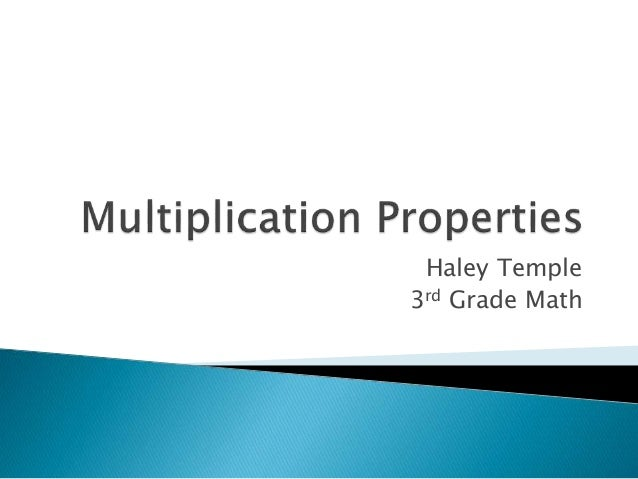Successfully reported this slideshow.
We use your LinkedIn profile and activity data to personalize ads and to show you more relevant ads. You can change your ad preferences anytime.Upcoming SlideShare
Loading in …5
×

# Multiplication Properties for 3rd Grade

This is important for 3rd grade students to become familiar with, This slide show will explain the differences in the four different multiplication properties

• Full Name
Comment goes here.

Are you sure you want to Yes No
Your message goes here• Login to see the comments

### Multiplication Properties for 3rd Grade

1. 1. Haley Temple 3rd Grade Math
2. 2.  When two numbers are multiplied together, the product will be the same regardless of the order  Ex. (4)(5)=(5)(4)
3. 3.  When three or more numbers are multiplied together, the product will be the same regardless of the grouping  Ex. (4x2) x 3 = 4 x (2x3)
4. 4.  When a number is multiplied by 1, the product will always be the number  Ex 8 x 1 = 8 150 x 1 = 150
5. 5.  The sum of two numbers times the third number is equal to the sum of each addend times the third number  Ex. 4 x (6 + 3) = 4x6 + 4x3